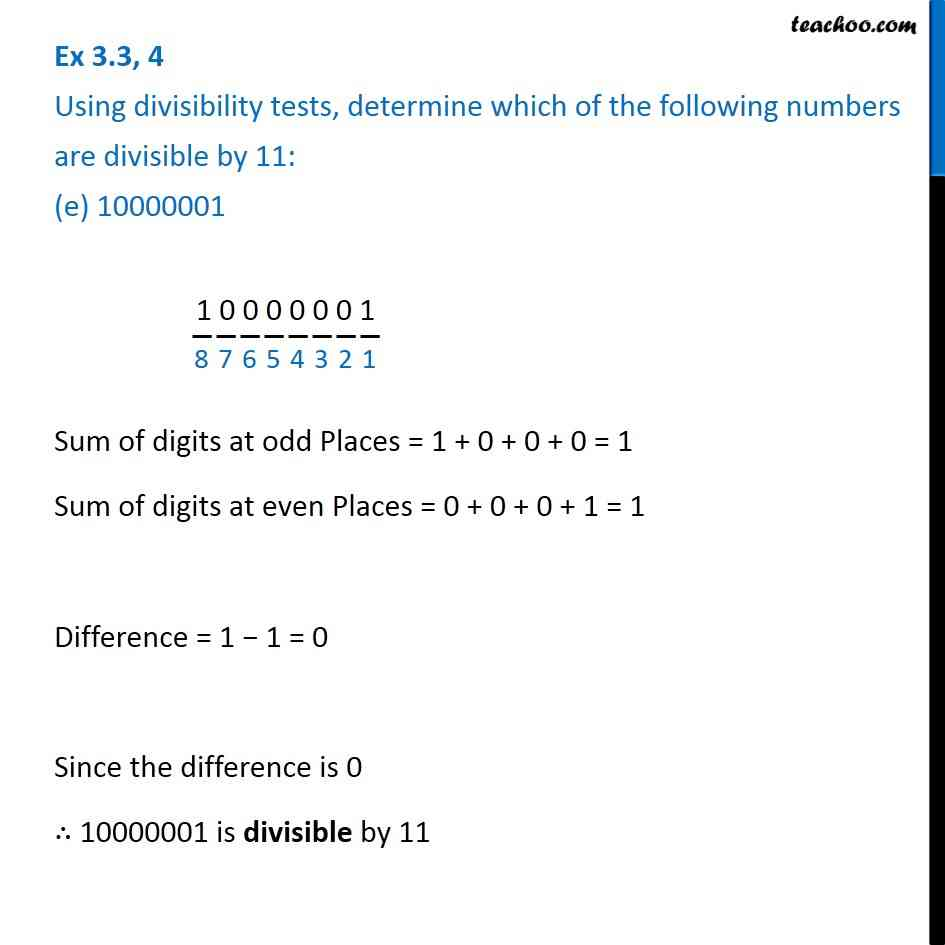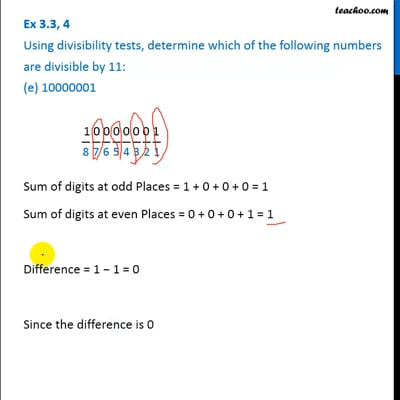Ex 3.3

Chapter 3 Class 6 Playing with Numbers
Serial order wiseThis video is only available for Teachoo black users

Introducing your new favourite teacher - Teachoo Black, at only ₹83 per month

### Transcript

Ex 3.3, 4 Using divisibility tests, determine which of the following numbers are divisible by 11: (e) 10000001 1 0 0 0 0 0 0 1 Sum of digits at odd Places = 1 + 0 + 0 + 0 = 1 Sum of digits at even Places = 0 + 0 + 0 + 1 = 1 Difference = 1 − 1 = 0 Since the difference is 0 ∴ 10000001 is divisible by 11